×#### Thank you for registering.

One of our academic counsellors will contact you within 1 working day.

Click to Chat

1800-1023-196

+91-120-4616500

CART 0

• 0

MY CART (5)

Use Coupon: CART20 and get 20% off on all online Study Material

ITEM
DETAILS
MRP
DISCOUNT
FINAL PRICE
Total Price: Rs.

There are no items in this cart.
Continue Shopping• Complete JEE Main/Advanced Course and Test Series
• OFFERED PRICE: Rs. 15,900
• View Details

```Chapter 22: Tabular Representation of Statistical Data Exercise – 22.1

Question: 1

What do you understand by the word “statistics” in:

(a) Singular form (B) Plural form

Solution:

The word statistics is used in both its singular and plural senses.

(a) In singular sense, statistics may be defined as the science of collection, presentation, analysis and interpretation of numerical data.

(b) In plural sense, statistics means numerical facts or observations collected with definite purpose.

For Example: Income and expenditure of persons in a particular locality, number of persons in a particular locality, number of males and females in a particular town are statistics.

Question: 2

Describe some fundamental characteristics of statistics.

Solution:

Fundamental characteristics of statistics

(1) A single observation does not form statistics. Statistics are a sum total of observations.

(2) Statistics are expressed quantitatively not qualitatively.

(3) Statistics are collected with definite purpose.

(4) Statistics in an experiment are comparable and can be classified into groups.

Question: 3

What are (1) primary data (2) secondary data? Which of the two - the primary or the secondary data - is more reliable and why?

Solution:

The word data means information statistical data and are of two types:

(1) Primary data

When an investigator collects data himself with a definite plan or design in his or her mind is called Primary Data.

(2) Secondary Data

Data which are not originally collected rather obtained from published or unpublished sources are called secondary data.

Secondary data are collected by an individual or an institution for some purpose and are used by someone else in another context.

Primary data are reliable and relevant because they are original in character and are collected by some individuals or by research bodies.

Question: 4

Why do we group data?

Solution:

The data obtained in original form are called raw data .Raw data does not give any useful information and is rather confusing to mind. Data is grouped so that it becomes understandable and can be interpreted. According to various characteristics groups are formed by us. After grouping the data, we are in position to make calculations of certain values which will help us in describing and analyzing the data.

Question: 5

Explain the meaning of the following terms:

(1) Variate

(2) Class-integral

(3) Class-size

(4) Class-mark

(5) Frequency

(6) Class limits

(7) True class limits

Solution:

(1) Variate

Any character that can vary from one individual to another is called variate.

(2) Class interval

In the data each group into which raw data is considered is called a class-interval.

(3) Class-size

The difference between the true upper limit and lower limit is called the class size of that class size.

(4) Class-mark

The middle value of the class is called as the class mark.(5) Frequency

The number of observations corresponding to class is called its frequency.

(6)Class limits

Each class is bounded by two figures ,called the class limits .The figures on the left side of the classes are called lower limits while figures on the right side are called upper limits.

(7)True class limits

If classes are inclusive.Eg: 15 - 19, 20 - 24, 25 - 29….

Then, true lower limit of class = Upper Limit of Class - 0.5

Eg:- True limits of the class is 15 - 19 are 14.5 and 19.5

But if classes are exclusive like 10 - 20, 20 - 30, 30 - 40…

Here class limits and true class limits are the same.

Question: 6

The ages of ten students of a group are given below. The ages have been recorded in years and months:

8 - 6, 9 - 0, 8 - 4, 9 - 3, 7-8, 8 - 11, 8 - 7, 9 - 2, 7 - 10, 8 - 8

(i) What is the lowest age?

(ii) What is the highest age?

(iii) Determine the range?

Solution:

The ages of ten students of a group are given below

8 - 6, 9 - 0, 8 - 4, 9 - 3, 7 - 8, 8 - 11, 8 -7, 9 - 2, 7 - 10, 8 - 8.

(1) Lowest age is 7 years 8 months

(2) Highest age is 9 years, 3months

(3) Range = Highest age-lowest age = 9 year, 3 months, 7 years, 8 months = 1 year 7 months

Question: 7

The monthly pocket money of six friends is given below:

Rs 45, Rs 30, Rs 40, Rs 25, Rs 45.

(i) What is the lowest pocket money?

(ii) What is the highest pocket money?

(iii) What is the range?

(iv) Arrange the amounts of pocket money in ascending order

Solution:

The monthly pocket money of six friends is given below:

Rs 45,Rs 30, Rs 40, Rs 25, Rs 45.

(1) Highest pocket money = Rs 50

(2) Lowest pocket money = Rs 25

(3) Range = 50 – 25 = 25

(4) The amounts of pocket money in an ascending order is: Rs 25, Rs 30, Rs 40, Rs 45, Rs 45, Rs 50.

Question: 8

Write the class -size in each of the following:

(1) 0 - 4, 5 - 9, 10 – 14

(2) 10 - 19, 20 - 29, 30 - 39

(3) 100 - 120, 120 - 140, 160 - 180

(4) 0 - 0.25, 0.25 - 00.50, 0.50 - 0.75

(5) 5 - 5.01, 5.01 - 5.02, 5.02 - 5.03.

Solution:

(1) 0 - 4, 5 - 9, 10 - 14

True class limits are 0.5 - 4.5, 4.5 - 9.5, 9.5 - 14.5

Therefore class size = 14.5 - 9.5 = 5

(2) 10 - 19, 20 - 29, 30 - 39

True class limitsà 19.5 - 19.5, 19.5 - 29.5, 29.5 - 29.5

Class size = 39.5 - 29.5 = 10

(3) 100 - 120, 120 - 140, 160 -180

Here the class limits and true class limits are the same

Therefore class size = 120 - 100 = 20

(4) 0 - 0.25, 0.25 - 00.50, 0.50 - 0.75

Here the class limits and true class limits are the same

Therefore class size = 0.25 – 0 = 0.25

(5) 5 - 5.01, 5.01 - 5.02, 5.02 - 5.03.

Here the class limits and true class limits are the same

Therefore class size = 5.01 - 5.0 = 0.01.

Question: 9

The final marks in mathematics of 30 students are as follows:

53, 61, 48, 60, 78, 68, 55, 100, 67, 0, 75, 88, 77, 37, 84, 58, 60, 48, 62, 56, 44, 58, 52, 64, 98, 59, 70, 39, 50, 60.

(i) Arrange these marks in ascending order 30 to 39 one group 40 to 49 second group etc.

(ii) What is the lowest score?

(iii) What is the highest score?

(iv) What is the range?

(v) If 40 is the pass mark how many failed?

(vi) How many have scored 75 or more?

(vii) Which observations between 50 and 60 have not actually appeared?

(viii) How many have scored less than 50?

Solution:

The final marks in mathematics of 30 students are as follows:

53, 61, 48, 60, 78, 68, 55, 100, 67, 0, 75, 88, 77, 37, 84, 58, 60, 48, 62, 56, 44, 58, 52, 64, 98, 59, 70, 39, 50, 60.

(i)

Group
Class
Observations

I
30 – 39
37, 39

II
40 – 49
44, 48, 48

III
50 – 59
50, 52, 53, 55, 56, 58, 58, 59

IV
60 – 69
60, 60, 60, 61, 62, 64, 67, 68

V
70 – 79
70, 55, 77, 78

VI
80 – 89
84, 88

VII
90 – 99
90, 98

VIII
100 – 109
100

(2) Highest score = 100

(3) Lowest score = 37

(4) Range = 100 - 37 = 63

(5) If 40 is the passing marks 2 students have failed

(6) 8 students have scored 75 or more

(7) Observation 51, 54, 57 between 50 and 60 has not actually appeared.

(8) 5 students have scored less than 50

Question: 10

The weights of new born babies are as follows: 2.3, 2.2, 2.1, 2.7, 2.6, 2.5, 3.0, 2.8, 2.8, 2.9, 3.1, 2.5, 2.8, 2.7, 2.9, 2. 4.

(i) Rearrange the weights in descending order.

(ii) What is the highest weight?

(iii) What is the lowest weight?

(iv) Determine the range?

(v) How many babies were born on that day?

(vi) How many babies weigh below 2.5 Kg?

(vii) How many babies weigh more than 2.8 Kg?

(viii) How many babies weigh 2.8 Kg?

Solution:

The weights of new born babies(in kg) are as follows

2.3, 2.2, 2.1, 2.7, 2.6, 2.5, 3.0, 2.8, 2.8, 2.9, 3.1, 2.5, 2.8, 2.7, 2.9, 2.4.

(i) The weights in descending order

3.1, 3.0, 2.9, 2.9, 2.8, 2.8, 2.7, 2.7, 2.6, 2.5, 2.5, 2.4, 2.3, 2.2, 2.1.

(ii) The highest weight = 3.1 Kg

(iii) The lowest weight = 2.1 Kg

(iv) Range = 3.1 - 2.1 = 1.0 Kg

(v) 15 babies were born on that particular day.

(vi) 4 babies weight below 2.5 Kg.

(vii) Weight more than 2.8 Kg are 4 babies.

(viii) Weightà 2 babies

Question: 11

The number of runs scored by a cricket player in 25 innings is as follows:

26, 35, 94, 48, 82, 105, 53, 0, 39, 42, 71, 0, 64, 15, 34, 15, 34, 6, 71, 0, 64, 15, 34, 15, 34, 67, 0, 42, 124, 84, 54, 48, 139, 64, 47

(i) Rearrange these runs in ascending order.

(ii) Determine the player, is highest score.

(iii) How many times did the player not score a run?

(iv) How many centuries did he score?

(v) How many times did he score more than 50 runs?

Solution:

The numbers of runs scored by a player in 25 innings are

26, 35, 94, 48, 82, 105, 53, 0, 39, 42, 71, 0, 64, 15, 34, 15, 34, 6, 71, 0, 64, 15, 34, 15, 34, 67, 0, 42, 124, 84, 54, 48, 139, 64, 47.

(i) Runs in an ascending order are

0, 0, 0, 0, 6, 15, 15, 15, 15, 26, 34, 34, 34, 34, 35, 39, 42, 42, 47, 48, 48, 53, 54, 64, 64, 64, 67, 71, 71, 82, 90, 124, 139.

(ii) The highest number = 139

(iii) The player did not score any runs 3 times

(iv) He scored 3 centuries.

(v) He scored more than 50 runs 12 times.

Question: 12

The class size of distribution is 25 and the first class-interval is 200 - 224. There are seven class-intervals.

(i) Write the class-intervals.

(ii) Write the class marks of each interval.

Solution:

Given

Class size = 25

First class interval = 200 - 224

(i) Seven class interval are:

200 - 240, 225 - 249, 250 - 274, 275 - 299, 300 - 324, 325 - 349,3 50 - 374.

(ii) Class mark = 200 - 224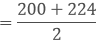= 424/2

= 212

Class mark = 225 - 249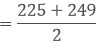= 479/2

= 237

Class mark = 250 - 274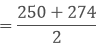= 524/2

= 287

Class mark = 300 - 324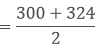= 624/2

= 312

Class mark = 325 - 349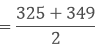= 674/2

= 337

Class mark = 350 - 374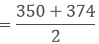= 724/2

= 362

Question: 13

Write the class size and class limits in each of the following:

(i) 104, 114, 124, 134, 144, 154 and 164.

(ii) 47, 52, 57, 62, 67, 72, 78, 82, 87, 92, 97, 102.

(iii) 12.5, 17.5, 22.5, 27.5, 32.5, 37.5, 42.5, 47.5.

Solution:

(i) 104, 114, 124, 134, 144, 154 and 164.

Class size = 114 – 104 = 10

Class Mark
Lower Class Limit
Upper Class Limit
Class Limit

104
104 −102 = 99
104 + 102 = 109
99 - 109

114
114 − 102 = 109
114 + 102 = 119
109 - 119

124
119
129
119 - 129

134
129
139
129 - 139

144
139
149
139 - 149

154
149
159
149 - 159

164
159
169
159 - 169

(ii)  47, 52, 57, 62, 67, 72, 78, 82, 87, 92, 97, 102.

Class size = 52 – 47 = 5

Class mark
Lower class limit
Upper class limit
Class limit

47
47 – 52 = 44.5
47 + 52 = 49.5
44.5 - 49.5

52
49.5
54.5
49.5 - 54.5

57
54.5
59.5
54.5 - 59.5

62
59.5
64.5
59.5 - 64.5

67
64.5
69.5
64.5 - 69.5

72
69.5
74.5
69.5 - 74.5

77
74.5
79.5
74.5 - 79.5

82
79.5
84.5
79.5 - 84.5

87
84.5
89.5
84.5 - 89.5

92
89.5
94.5
89.5 - 94.5

97
94.5
99.5
94.5 - 99.5

102
99.5
104.5
99.5 - 104.5

(iii) 12.5, 17.5, 22.5, 27.5, 32.5, 37.5, 42.5, 47.5.

Class size = 17.5 - 12.5 = 5

Class Mark
Lower Class Limit
Upper Class Limit
Class Limit

12.5
12.5 - 2.5 = 10
12.5 + 2.5 = 15
15-Oct

17.5
17.5 - 2.5 = 15
17.5 + 2.5 = 20
15 - 20

22.5
22.5 - 2.5 = 20
22.5 + 2.5 = 25
20 - 25

27.5
27.5 - 2.5 = 25
27.5 + 2.5 = 30
25 - 30

32.5
32.5 - 2.5 = 30
32.5 + 2.5 = 35
30 - 35

37.5
37.5 - 2.5 = 35
37.5 + 2.5 = 40
35 - 40

42.5
42.5 - 2.5 = 40
42.5 + 2.5 = 45
40 - 45

47.5
47.5 - 2.5 = 45
47.5 + 2.5 = 50
45 - 50

Question: 14

Following data gives the number of children in 40 families:

1, 2, 6, 5, 1, 5, 1, 3, 2, 6, 2, 3, 4, 2, 0, 0, 4, 4, 3, 2, 2, 0, 0, 1, 2, 2, 4, 3, 2, 1, 0, 5, 1, 2, 4, 3, 4, 1, 6, 2, 2.

Represent it in the form of a frequency distribution.

Solution:

Number of Children
Tally Marks
Number of Families

0
||||
5

1
|||| ||
7

2
|||| |||| ||
12

3
||||
5

4
|||| |
6

5
|||
3

6
|||
3

Question: 15

Marks scored by 40 students of class IX in mathematics are given below:

81, 55, 68, 79, 85, 43, 29, 68, 54, 73, 47, 35, 72, 64, 95, 44, 50, 77, 64, 35, 79, 52, 45, 54, 70, 83, 62, 64, 72, 92, 84, 76, 63, 43, 54, 38, 73, 68, 52, 54.

Prepare a frequency distribution with class size of 10 marks.

Solution:

Marks
Tally Marks
Frequency

20 - 30
|
1

30 - 40
|||
3

40-50
||||
5

50-60
|||| |||
8

60-70
|||| |||
8

70-80
|||| ||||
9

80-90
||||
4

Total = 40

Question: 16

Heights (in cm) of 30 students of class IX are given below:

155, 158, 154, 158, 160, 148, 149, 150, 153, 159, 161, 148, 157, 153, 157, 162, 159, 151,  154,  156 , 152 , 156 , 160,  152,  147,  155,  163,  155 , 157 , 153.

Prepare a frequency distribution table with 160 - 164 as one of the class intervals.

Solution:

Height (in cm)
Tally marks
Frequency

800 – 810
|||
3

810 – 820
||
2

820 – 830
||
1

830 – 840
|||| |||
8

840 – 850
||||
5

850 – 860
|
1

860 – 870
|||
3

870 – 880
|
1

880 – 890
|
1

890 – 900
||||
5

Total = 30

Question: 17

The daily maximum temperatures (in degree Celsius) recorded in a certain city during the month of November are as follows:

25.8, 24.5, 25.6, 20.7, 21.8, 20.5, 20.6, 20.9, 22.3, 22.7, 23.1, 22.8, 22.9, 21.7, 21.3, 20.5, 20.9, 23.1, 22.4,  21.5, 22.7, 22.8, 22.0, 23.9, 24.7, 22.8, 23.8, 24.6, 23.9, 21.1.

Represent the data in the form of a frequency distribution table with class size 11°C.

Solution:

Maximum Temperature(in degree Celsius)
Tally Marks
Frequency

20.0 – 21.0
|||| |
6

21.0 – 22.0
||||
5

22.0 – 23.0
|||| ||||
9

23.0 – 24.0
||||
5

24.0 – 25.0
|||
3

25.0 – 26.0
||
2

Total = 30

Question: 18

Construct a frequency table with equal class intervals from the following data on the monthly wages (in rupees) of 28 laborers working in a factory, taking one of the class intervals as 210 – 230 (230 not included).

220, 268, 258, 242, 210, 268, 272, 242, 311, 290, 300, 320, 319, 304, 302, 218, 306, 292, 254, 278, 210, 240, 280, 316, 306, 215, 256, 236.

Solution:

Monthly Wages (in rupees)
Tally marks
Frequency

210 – 230
||||
4

230 – 250
||||
4

250 – 270
||||
5

270 – 290
|||
3

290 – 310
|||| ||
7

310.0 – 330.0
||||
5

Total = 28

Question: 19

The daily minimum temperatures in degree Celsius recorded in a certain arctic region are as follows:

-12.5, -10.8, -18.6, -8.4-10.8, -4.2, -4.8, -6.7, -13.2, -11.8, -2.3, -1.2,

-2.6, 0, 2.4, 0, 3.2, 2.7, 3.4, 0, -2.4, -2.4, 0, 3.2, 2.7, 3.4, 0, -2.4, -5.8, -8.9, -14.6, -12.3,

-11.5, -7.8, -2.9.

Represent them as frequency distribution table taking -19.9 to -15 as the first class interval.

Solution:

Since first class interval is -19.9 to -15, frequency distribution with lower limit included and upper limit excluded is:

Temperature
Tally Marks
Frequency

- 19.9 to – 15
||
2

- 15 to – 10.1
|||| ||
7

-10.1 to – 5.2
||||
5

- 5.2 to – 0.3
||||
4

- 0.3 to – 4.6
|||| |||| |||| ||
17

Total = 35

Question: 20

The blood groups of 30 students of class VIII are recorded as follows:

A, B, O, O, AB, O, A, O, B, A, O, B, A, O, O, A, AB, O, A, A, O, O, AB, B, A, O, B, A, B, O

Represent this data in the form of a frequency distribution table .Find out which is the most common and which is the most rarest blood group among these students.

Solution:

Here 9 students have blood group A, 6 as B, 3 as AB and 12 as O

So the table representing the data is as follows:

Blood Group
Number of Students

A
9

B
6

AB
3

O
12

Total
30

As 12 students have their blood group O and 3 students have their blood group as AB. Therefore the most common blood group is O and the rarest blood group is AB.

Question: 21

Three coins were tossed 30 times. Each time the number of heads occurring was noted down as follows:

0, 1, 2, 2, 1, 2, 3, 1, 3, 0

1, 3, 1, 1, 2, 2, 0, 1, 2, 1

3, 0, 1, 1, 2, 3, 2, 2, 0

Prepare a frequency distribution table for the data given above.

Solution:

By observing the data given above, the following frequency table can be constructed:

Frequency

0
6

1
10

2
9

3
5

Total
30

Question: 22

Thirty children were asked about the number of hours they watched TV programes in the previous week. The results were found as follows:

1, 6, 2, 3, 5, 12, 5, 8, 4, 8

10, 3, 4, 12, 2, 8, 15, 1, 17, 6

3, 2, 8, 5, 9, 6, 8, 7, 14, 2.

(i) Make a frequency distribution table for this data, taking class width 5 and one of the class intervals as 5 - 10.

(ii) How many children watched television for 15 or more hours a week.

Solution:

(i) Class intervals will be 0 - 5, 5 - 10, 10 - 15 …

The grouped frequency distribution table is as follows:

Hours
Number of children

0 – 5
10

5 – 10
13

10 – 15
5

15 – 20
2

Total
30

(ii) The number of children who watched TV for 15 or more hours a week is 2(i.e number of children in class interval 15 - 20).

```### Course Features

• 728 Video Lectures
• Revision Notes
• Previous Year Papers
• Mind Map
• Study Planner
• NCERT Solutions
• Discussion Forum
• Test paper with Video Solution